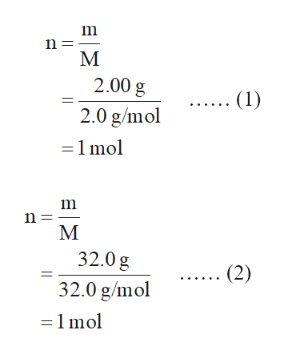# Use 1 decimal point for all atomic masses. 2.00 g of H2(g) are reacted with 32.0 g of O2(g) by the following reaction 2H2(g) + O2(g) --> 2H2O(g)What is the limiting reagent?H2(g)O2(g)    Based on the limiting reagent, fill in the following ICE Table using moles. Enter any zero values as 0.00.  H2O2H2OInitial   Change   Final    How much of the excess reagent remains (in grams)?

Question
12 views

Use 1 decimal point for all atomic masses. 2.00 g of H2(g) are reacted with 32.0 g of O2(g) by the following reaction

 2H2(g) + O2(g) --> 2H2O(g) What is the limiting reagent? H2(g) O2(g) Based on the limiting reagent, fill in the following ICE Table using moles. Enter any zero values as 0.00.

 H2 O2 H2O Initial Change Final

How much of the excess reagent remains (in grams)?

check_circle

Step 1

The balanced reaction is written below.

2H2 (g) + O2 (g) → 2H2O (g)

The number of moles of H2 is calculated in equation (1).

The number of moles of O2 is calculated in equation (2).help_outlineImage Transcriptionclosen М 2.00 g .... (1) 2.0 g/mol 1mol m n М 32.0g 32.0 g/mol (2) 1 mol fullscreen
Step 2

According to the balanced chemical reaction, 2 mol of H2 requires 1 mol of O2. Thus, the moles of oxygen required by 1 mol of H2 is calculated below.

Step 3

The number of moles of oxygen actually present is 1 mol. Thus, O2 is pre...

### Want to see the full answer?

See Solution

#### Want to see this answer and more?

Solutions are written by subject experts who are available 24/7. Questions are typically answered within 1 hour.*

See Solution
*Response times may vary by subject and question.
Tagged in

### Chemistry